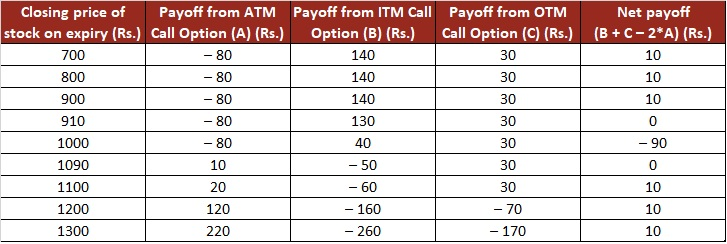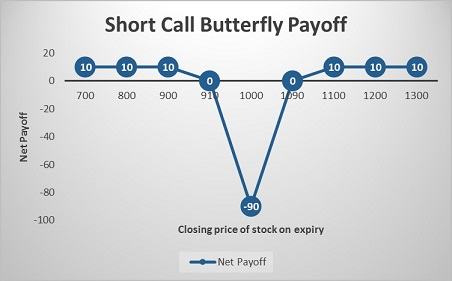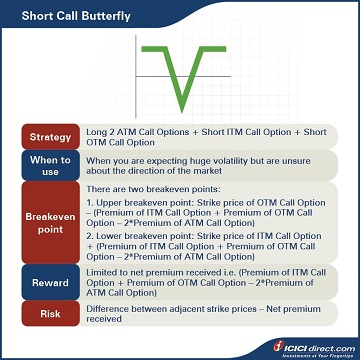Abhinav’s new manager tells him that she is expecting huge volatility in the price of a stock, ABC Ltd., but has a neutral view on the direction. She asks Abhinav to pick out an Options strategy that would be beneficial in this situation. After some research, Abhinav suggests Short Call Butterfly.

## Short Call Butterfly

A Short Call Butterfly is a three-legged Options strategy that involves selling one ITM Call Option, buying two ATM Call Options of the same strike and selling one OTM Call Option, where the three strike prices have an equal difference.

This strategy is used when the trader is neutral on the direction of the market, but is very bullish on the movement of the prices of the underlying. Since Simran believes that there will be huge volatility in the prices of the underlying, either on the upside or downside, she acknowledges that Abhinav’s suggestion is a good one.

• This strategy will give maximum profit when the spot prices reach either the lower or higher strike prices of the Call Options.

Strategy: Long 2 ATM Call Options (Leg 1) + Short ITM Call Option (Leg 2) + Short OTM Call Option (Leg 3)

When to use: When you are expecting huge volatility but are unsure about the direction of the market

Breakeven: There are two breakeven points:

1. Upper breakeven point: Strike price of OTM Call Option – (Premium of ITM Call Option + Premium of OTM Call Option – Premium of ATM Call Option)

2. Lower breakeven point: Strike price of ITM Call Option + (Premium of ITM Call Option + Premium of OTM Call Option – Premium of ATM Call Option)

 Did you know?  A Short Call Butterfly is a great strategy to employ when you are confident that an underlying security may move in either direction. This is an advanced strategy that limits the risk to reward ratio.

### Let’s understand the Short Call Butterfly strategy with an example:

Assume that the spot price of ABC Ltd. is Rs. 1,000. Abhinav sells an ABC Ltd. ITM Call of strike price Rs. 900 at Rs. 140, sells an OTM Call of strike price Rs. 1,100 at Rs. 30, and buys 2 ATM Call Options of strike price Rs. 1,000 at Rs. 80. He receives a total premium of Rs. 140 + Rs. 30 – Rs. 2*80 = Rs. 10, and this will be the maximum profit.

### Let’s take a look at the cash flow in various scenarios:If the stock closes at Rs. 800 on expiry: All the three legs expire OTM Let’s understand the payoff in various scenarios. It will give you a fair idea of how we have arrived at the above values.

Leg 1: Premium paid on the two ATM Call Options of strike price Rs. 1000 = 2*80 = Rs. 160

Premium received on the two ATM Call Options of strike price Rs. 1000 = 2* Max {0, (Spot price –  Strike price)} = 2* Max {0, (800 – 1000)} = 2*Max (0, – 200) = 0

So, the payoff from the two ATM Call Options = Premium received – Premium paid = 0 – 160 = –Rs. 160

Leg 2: Premium received on the ITM Call Option of strike price Rs. 900 = Rs. 140

Premium paid on ITM Call Option of strike price Rs. 900 at expiry = Max {0, (Spot price – Strike price)} = Max {0, (800 – 900)} = Max (0, – 100) = 0

So, the payoff from the ITM Call Option = Premium received – Premium paid = 140 – 0 = Rs. 140

Leg 3: Premium received on the OTM Call Option of strike price Rs.1100 = Rs. 30

Premium paid on OTM Call Option of strike price Rs. 1100 at expiry = Max {0, (Spot price – Strike price)} = Max {0, (800 – 1100)} = Max (0, – 300) = 0

So, the Payoff from the OTM Call Option = Premium received – Premium paid = 30 – 0 = Rs. 30

Net Payoff = Payoff from two ATM Call Options + Payoff from ITM Call Option + Payoff from OTM Call Option = (– 160) + 140 + 30 = Rs. 10

### If the stock closes at Rs. 1090 on expiry: Leg 1 and leg 2 expire ITM while leg 3 expires OTM

Leg 1: Premium paid on the two ATM Call Options of strike price Rs. 1000 = 2*80 = Rs. 160

Premium received on the two ATM Call Options of strike price Rs. 1000 = 2* Max {0, (Spot price –  Strike price)} = 2* Max {0, (1090 – 1000)} = 2*Max (0, 90) = 2*90 = Rs. 180

So, the payoff from the two ATM Call Options = Premium received – Premium paid = 180 – 160 = Rs. 20

Leg 2: Premium received on the ITM Call Option of strike price Rs. 900 = Rs. 140

Premium paid on ITM Call Option of strike price Rs. 900 at expiry = Max {0, (Spot price – Strike price)} = Max {0, (1090 – 900)} = Max (0, 190) = Rs. 190

So, the payoff from the ITM Call Option = Premium received – Premium paid = 140 – 190 = – Rs. 50

Leg 3: Premium received on the OTM Call Option of strike price Rs.1100 = Rs. 30

Premium paid on the OTM Call Option of strike price Rs. 1100 at expiry = Max {0, (Spot price – Strike price)} = Max {0, (1090 – 1100)} = Max (0, – 10) = 0

So, the Payoff from the OTM Call Option = Premium received – Premium paid = 30 – 0 = Rs. 30

Net Payoff = Payoff from two ATM Call Options + Payoff from ITM Call Option + Payoff from OTM Call Option = 20 + (– 50) + 30 = 0

### If the Stock closes at Rs. 1200 on expiry: All three legs expire ITM

Leg 1: Premium paid on the two ATM Call Options of strike price Rs. 1000 = 2*80 = Rs. 160

Premium received on the two ATM Call Options of strike price Rs. 1000 = 2* Max {0, (Spot price –  Strike price)} = 2* Max {0, (1200 – 1000)} = 2*Max (0, 200) = 2*200 = Rs. 400

So, the payoff from the two ATM Call Options = Premium received – Premium paid = 400 – 160 = Rs. 240

Leg 2: Premium received on the ITM Call Option of strike price Rs. 900 = Rs. 140

Premium paid on ITM Call Option of strike price Rs. 900 at expiry = Max {0, (Spot price – Strike price)} = Max {0, (1200 – 900)} = Max (0, 300) = Rs. 300

So, the payoff from the ITM Call Option = Premium received – Premium paid = 140 – 300 = – Rs. 160

Leg 3: Premium received on the OTM Call Option of strike price Rs.1100 = Rs. 30

Premium paid on OTM Call Option of strike price Rs. 1100 at expiry = Max {0, (Spot price – Strike price)} = Max {0, (1200 – 1100)} = Max (0, 100) = Rs.100

So, the Payoff from the OTM Call Option = Premium received – Premium paid = 30 – 100 = – Rs. 70

Net Payoff = Payoff from two ATM Call Options + Payoff from ITM Call Option + Payoff from OTM Call Option = 240 + (– 160) + (– 70) = Rs. 10

Additional Read: What are Options? How do they work? Types and Features of Option## Summary• A Short Call Butterfly is a three-legged Options strategy that involves selling one ITM Call Option, buying two ATM Call Options and selling one OTM Call Option where the three strike prices have an equal difference.
• This strategy is used when the trader is neutral on the direction of the market but is very bullish on the movement of the prices of the underlying.
• Breakeven: There are two breakeven points:
• Upper breakeven point: Strike price of OTM Call Option – (Premium of ITM Call Option + Premium of OTM Call Option – Premium of ATM Call Option)
• Lower breakeven point: Strike price of ITM Call Option + (Premium of ITM Call Option + Premium of OTM Call Option – Premium of ATM Call Option)